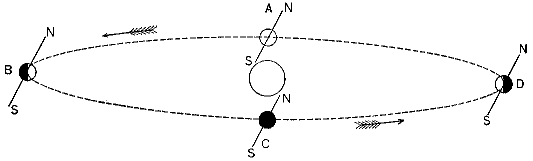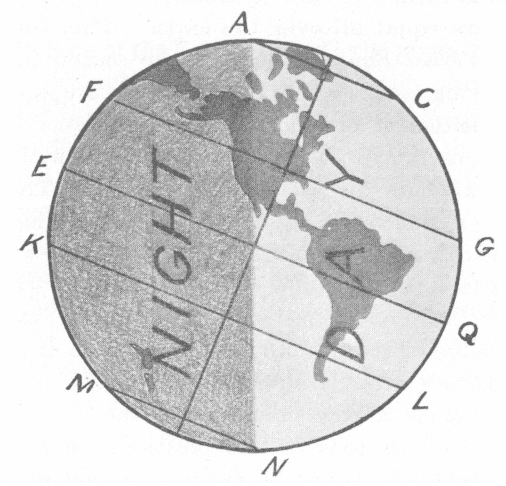## 2. Annual Motion of the Earth round the Sun

We must now explain the motion of the earth in its orbit round the sun. This is called its annual motion, because it takes a year to complete one revolution.We cannot draw a figure which shall represent the earth and its orbit in anything like their true proportions, because the diameter of the orbit is more than 20,000 times that of the earth itself. So we have to draw figures, as before, on two very different scales. Figure 16 shows the orbit of the earth seen nearly edgewise. The plane containing this orbit is called the plane of the ecliptic. On a true scale the earth in this figure would be an invisible dot, so we make it larger, and then represent it on a still larger scale in figure 17.In figure 16 the direction of the axis is shown by the inclined line NS.

An important law of the earth’s motion is this: As the earth moves round the sun, the direction of its axis remains almost unchanged.

This direction is not quite perpendicular to the ecliptic, but is inclined to the perpendicular by 23½°, or a little more than one fourth of a right angle. This angle is called the obliquity of the ecliptic, because it is equal to the angle which the plane of the equator makes with the plane of the ecliptic.